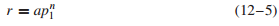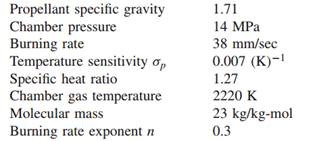### Create an Account

Home / Questions / What would the area ratio Ab At in Problem 1 be if the pressure were increased by 10 Use ...

# What would the area ratio Ab At in Problem 1 be if the pressure were increased by 10 Use curve from Problem 2 Problem 2 Plot the burning rate against chamber pressure for the motor in Problem

What would the area ratio Ab /At in Problem 1 be if the pressure were increased by 10%? (Use curve from Problem 2.)

Problem 2

Plot the burning rate against chamber pressure for the motor in Problem 1 using Eq. 12–5 between chamber pressures of 11 and 20 MPa.Problem 1

What is the ratio of the burning area to the nozzle throat area for a solid propellant motor with these characteristics? Also, calculate the temperature coefficient (a) and the temperature sensitivity of pressure (πK).Jul 23 2020 View more View LessSubscribe To Get Solution